# Binding Energy Physics Notes | EduRev

## Physics : Binding Energy Physics Notes | EduRev

The document Binding Energy Physics Notes | EduRev is a part of the Physics Course Modern Physics for IIT JAM.
All you need of Physics at this link: Physics

The more tightly bound a system is, the stronger the forces that hold it together and the greater the energy required to pull it apart. We can therefore learn about nuclear forces by examining how tightly bound the nuclei are. We define the binding energy (BE) of a nucleus to be the energy required to completely disassemble it into separate protons and neutrons. We can determine the BE of a nucleus from its rest mass. The two are connected through Einstein’s famous relationship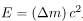. A bound system has a smaller mass than its separate constituents; the more tightly the nucleons are bound together, the smaller the mass of the nucleus.
Imagine pulling a nuclide. Work done to overcome the nuclear forces holding the nucleus together puts energy into the system. By definition, the energy input equals the binding energy BE. The pieces are at rest when separated, and so the energy put into them increases their total rest mass compared with what it was when they were glued together as a nucleus. That mass increase is thus m = BE/c2. This difference in mass is known as mass defect. It implies that the mass of the nucleus is less than the sum of the masses of its constituent protons and neutrons. A nuclide Ax has Z protons and N neutrons, so that the difference in mass is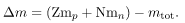Thus,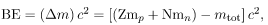where mtot is the mass of the nuclide Ax, mp is the mass of a proton, and mn is the mass of a neutron. Traditionally, we deal with the masses of neutral atoms. To get atomic masses into the last equation, we first add Z electrons to mtot , which gives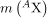, the atomic mass of the nuclide. We then add Z electrons to the Z protons, which gives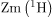, or z times the mass of a hydrogen atom. Thus the binding energy of a nuclide Ax is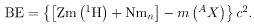The atomic masses can be found in Appendix A, most conveniently expressed in unified atomic mass units u (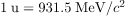). BE is thus calculated from known atomic masses.
Work done to pull a nucleus apart into its constituent protons and neutrons increases the mass of the system. The work to disassemble the nucleus equals its binding energy BE. A bound system has less mass than the sum of its parts, especially noticeable in the nuclei, where forces and energies are very large.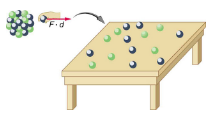Things Great and Small
Nuclear Decay Helps Explain Earth’s Hot Interior
A puzzle created by radioactive dating of rocks is resolved by radioactive heating of Earth’s interior. This intriguing story is another example of how small-scale physics can explain large-scale phenomena.
Radioactive dating plays a role in determining the approximate age of the Earth. The oldest rocks on Earth solidified about 3.5109 years ago—a number determined by uranium-238 dating. These rocks could only have solidified once the surface of the Earth had cooled sufficiently. The temperature of the Earth at formation can be estimated based on gravitational potential energy of the assemblage of pieces being converted to thermal energy. Using heat transfer concepts discussed in Thermodynamics it is then possible to calculate how long it would take for the surface to cool to rock-formation temperatures. The result is about 109 years. The first rocks formed have been solid for 3.5109 years, so that the age of the Earth is approximately 4.5109 years. There is a large body of other types of evidence (both Earth-bound and solar system characteristics are used) that supports this age. The puzzle is that, given its age and initial temperature, the center of the Earth should be much cooler than it is today.
The center of the Earth cools by well-known heat transfer methods. Convection in the liquid regions and conduction move thermal energy to the surface, where it radiates into cold, dark space. Given the age of the Earth and its initial temperature, it should have cooled to a lower temperature by now. The blowup shows that nuclear decay releases energy in the Earth’s interior. This energy has slowed the cooling process and is responsible for the interior still being molten.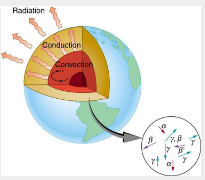We know from seismic waves produced by earthquakes that parts of the interior of the Earth are liquid. Shear or transverse waves cannot travel through a liquid and are not transmitted through the Earth’s core. Yet compression or longitudinal waves can pass through a liquid and do go through the core. From this information, the temperature of the interior can be estimated. As noticed, the interior should have cooled more from its initial temperature in the 4.5109 years since its formation. In fact, it should have taken no more than about 109 years to cool to its present temperature. What is keeping it hot? The answer seems to be radioactive decay of primordial elements that were part of the material that formed the Earth.
Nuclides such as 238U and 40K have half-lives similar to or longer than the age of the Earth, and their decay still contributes energy to the interior. Some of the primordial radioactive nuclides have unstable decay products that also release energy— 238U  has a long decay chain of these. Further, there were more of these primordial radioactive nuclides early in the life of the Earth, and thus the activity and energy contributed were greater then (perhaps by an order of magnitude). The amount of power created by these decays per cubic meter is very small. However, since a huge volume of material lies deep below the surface, this relatively small amount of energy cannot escape quickly. The power produced near the surface has much less distance to go to escape and has a negligible effect on surface temperatures.
A final effect of this trapped radiation merits mention. Alpha decay produces helium nuclei, which form helium atoms when they are stopped and capture electrons. Most of the helium on Earth is obtained from wells and is produced in this manner. Any helium in the atmosphere will escape in geologically short times because of its high thermal velocity.
What patterns and insights are gained from an examination of the binding energy of various nuclides? First, we find that BE is approximately proportional to the number of nucleons A in any nucleus. About twice as much energy is needed to pull apart a nucleus like 24Mg compared with pulling apart 12C, for example. To help us look at other effects, we divide BE by A and consider the binding energy per nucleon, BE/A. The graph of BE/A reveals some very interesting aspects of nuclei. We see that the binding energy per nucleon averages about 8 MeV, but is lower for both the lightest and heaviest nuclei. This overall trend, in which nuclei with A equal to about 60 have the greatest BE/A and are thus the most tightly bound, is due to the combined characteristics of the attractive nuclear forces and the repulsive Coulomb force. It is especially important to note two things—the strong nuclear force is about 100 times stronger than the Coulomb force, and the nuclear forces are shorter in range compared to the Coulomb force. So, for low-mass nuclei, the nuclear attraction dominates and each added nucleon forms bonds with all others, causing progressively heavier nuclei to have progressively greater values of BE/A . This continues up to A≈60, roughly corresponding to the mass number of iron. Beyond that, new nucleons added to a nucleus will be too far from some others to feel their nuclear attraction. Added protons, however, feel the repulsion of all other protons, since the Coulomb force is longer in range. Coulomb repulsion grows for progressively heavier nuclei, but nuclear attraction remains about the same, and so BE/A becomes smaller. This is why stable nuclei heavier than A ≈40 have more neutrons than protons. Coulomb repulsion is reduced by having more neutrons to keep the protons farther apart.
A graph of average binding energy per nucleon, BE/A , for stable nuclei. The most tightly bound nuclei are those with A near 60, where the attractive nuclear force has its greatest effect. At higher A s, the Coulomb repulsion progressively reduces the binding energy per nucleon, because the nuclear force is short ranged. The spikes on the curve are very tightly bound nuclides and indicate shell closures.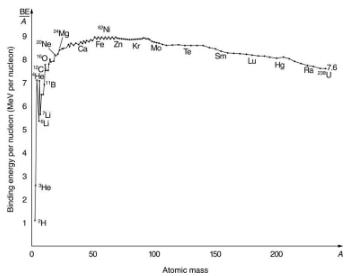The nuclear force is attractive and stronger than the Coulomb force, but it is short ranged. In low-mass nuclei, each nucleon feels the nuclear attraction of all others. In larger nuclei, the range of the nuclear force, shown for a single nucleon, is smaller than the size of the nucleus, but the Coulomb repulsion from all protons reaches all others. If the nucleus is large enough, the Coulomb repulsion can add to overcome the nuclear attraction.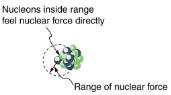There are some noticeable spikes on the BE/A graph, which represent particularly tightly bound nuclei. These spikes reveal further details of nuclear forces, such as confirming that closed-shell nuclei (those with magic numbers of protons or neutrons or both) are more tightly bound. The spikes also indicate that some nuclei with even numbers for Z and N, and with Z=N, are exceptionally tightly bound. This finding can be correlated with some of the cosmic abundances of the elements. The most common elements in the universe, as determined by observations of atomic spectra from outer space, are hydrogen, followed by 4He, with much smaller amounts of 12C and other elements. It should be noted that the heavier elements are created in supernova explosions, while the lighter ones are produced by nuclear fusion during the normal life cycles of stars, as will be discussed in subsequent chapters. The most common elements have the most tightly bound nuclei. It is also no accident that one of the most tightly bound light nuclei is 4He, emitted in α decay.

What Is BE/A for an Alpha Particle?

Calculate the binding energy per nucleon of {}^{4}\text{He}, the \alpha particle.
Strategy

To find BE/A, we first find BE using the Equation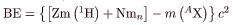and then divide by A. This is straightforward once we have looked up the appropriate atomic masses
Solution
The binding energy for a nucleus is given by the equation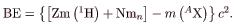For 4He, we have Z=N=2; thus,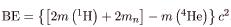Appendix A gives these masses as m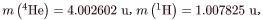Thus,
BE = (0.030378u)c2

Noting that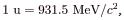we find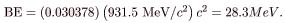Since A=4, we see that \text{BE}/A is this number divided by 4, or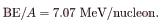Discussion
This is a large binding energy per nucleon compared with those for other low-mass nuclei, which have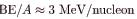. This indicates that 4He is tightly bound compared with its neighbors on the chart of the nuclides. You can see the spike representing this value of BE/A for 4He. This is why 4He is stable. Since 4He is tightly bound, it has less mass than other A=4 nuclei and, therefore, cannot spontaneously decay into them. The large binding energy also helps to explain why some nuclei undergo α decay. Smaller mass in the decay products can mean energy release, and such decays can be spontaneous. Further, it can happen that two protons and two neutrons in a nucleus can randomly find themselves together, experience the exceptionally large nuclear force that binds this combination, and act as a 4He unit within the nucleus, at least for a while. In some cases, the 4He escapes, and α decay has then taken place.
There is more to be learned from nuclear binding energies. The general trend in BE/A is fundamental to energy production in stars, and to fusion and fission energy sources on Earth, for example. This is one of the applications of nuclear physics covered in Medical Applications of Nuclear Physics. The abundance of elements on Earth, in stars, and in the universe as a whole is related to the binding energy of nuclei and has implications for the continued expansion of the universe.
Problem-Solving Strategies
For Reaction And Binding Energies and Activity Calculations in Nuclear Physics
1. Identify exactly what needs to be determined in the problem (identify the unknowns). This will allow you to decide whether the energy of a decay or nuclear reaction is involved, for example, or whether the problem is primarily concerned with activity (rate of decay).
2. Make a list of what is given or can be inferred from the problem as stated (identify the knowns).
3. For reaction and binding-energy problems, we use atomic rather than nuclear masses. Since the masses of neutral atoms are used, you must count the number of electrons involved. If these do not balance (such as in β+ decay), then an energy adjustment of 0.511 MeV per electron must be made. Also note that atomic masses may not be given in a problem; they can be found in tables.
4. For problems involving activity, the relationship of activity to half-life, and the number of nuclei given in the equation R = 0.693N/t1/2 can be very useful. Owing to the fact that number of nuclei is involved, you will also need to be familiar with moles and Avogadro’s number.
5. Perform the desired calculation; keep careful track of plus and minus signs as well as powers of 10.
6. Check the answer to see if it is reasonable: Does it make sense? Compare your results with worked examples and other information in the text. (Heeding the advice in Step 5 will also help you to be certain of your result.) You must understand the problem conceptually to be able to determine whether the numerical result is reasonable.
Section Summary
The binding energy (BE) of a nucleus is the energy needed to separate it into individual protons and neutrons. In terms of atomic masses,
where m(1H) is the mass of a hydrogen atom, m(4X) is the atomic mass of the nuclide, and mn is the mass of a neutron. Patterns in the binding energy per nucleon, BE/A, reveal details of the nuclear force. The larger the BE/A, the more stable the nucleus.
Conceptual Questions
Why is the number of neutrons greater than the number of protons in stable nuclei having Agreater than about 40, and why is this effect more pronounced for the heaviest nuclei?
Problems & Exercises
2H is a loosely bound isotope of hydrogen. Called deuterium or heavy hydrogen, it is stable but relatively rare—it is 0.015% of natural hydrogen. Note that deuterium has Z=N , which should tend to make it more tightly bound, but both are odd numbers. Calculate BE/A, the binding energy per nucleon, for 2H and compare it with the approximate value obtained from the graph.
112 MeV, consistent with graph
56Fe is among the most tightly bound of all nuclides. It is more than 90% of natural iron. Note that 56Fe has even numbers of both protons and neutrons. Calculate BE/A, the binding energy per nucleon, for 56Fe and compare it with the approximate value obtained from the graph.
209Bi is the heaviest stable nuclide, and its BE/A is low compared with medium-mass nuclides. Calculate BE/A , the binding energy per nucleon, for 209Bi and compare it with the approximate value obtained from the graph in [link].
7.848 MeV, consistent with graph
(a) Calculate BE/A for 235U , the rarer of the two most common uranium isotopes.
(b) Calculate BE/A for 238U. (Most of uranium is 238U.) Note that 238U has even numbers of both protons and neutrons. Is the BE/A of 238U significantly different from that of 235U ?
(a) Calculate BE/A for 235U. Stable and relatively tightly bound, this nuclide is most of natural carbon.

(b) Calculate BE/A for 14C. Is the difference in BE/A between 12C and 14C significant? One is stable and common, and the other is unstable and rare.
(a) 7.680 MeV, consistent with graph
(b) 7.520 MeV, consistent with graph. Not significantly different from value for 12C, but sufficiently lower to allow decay into another nuclide that is more tightly bound.
The fact that BE/A is greatest for A near 60 implies that the range of the nuclear force is about the diameter of such nuclides.
(a) Calculate the diameter of an A=60 nucleus.
(b) Compare BE/A for 58Ni and 90Sr. The first is one of the most tightly bound nuclides, while the second is larger and less tightly bound.
The purpose of this problem is to show in three ways that the binding energy of the electron in a hydrogen atom is negligible compared with the masses of the proton and electron.
(a) Calculate the mass equivalent in u of the 13.6-eV binding energy of an electron in a hydrogen atom, and compare this with the mass of the hydrogen atom obtained from Appendix A.
(b) Subtract the mass of the proton given in [link] from the mass of the hydrogen atom given in Appendix A. You will find the difference is equal to the electron’s mass to three digits, implying the binding energy is small in comparison.
(c) Take the ratio of the binding energy of the electron (13.6 eV) to the energy equivalent of the electron’s mass (0.511 MeV).
(d) Discuss how your answers confirm the stated purpose of this problem.
(a) 1.4610-8 u  vs. 1.007825 u for 1H
(b) 0.000549 u
(c) 2.6610-5
Unreasonable Results
A particle physicist discovers a neutral particle with a mass of 2.02733 u that he assumes is two neutrons bound together.
(a) Find the binding energy.
(c) What assumptions are unreasonable or inconsistent?
(a) -9.315MeV
(b) The negative binding energy implies an unbound system.
(c) This assumption that it is two bound neutrons is incorrect.
Offer running on EduRev: Apply code STAYHOME200 to get INR 200 off on our premium plan EduRev Infinity!

## Modern Physics for IIT JAM

20 videos|44 docs|15 tests

,

,

,

,

,

,

,

,

,

,

,

,

,

,

,

,

,

,

,

,

,

;